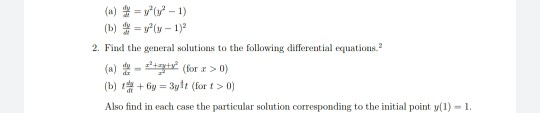Home / Answered Questions / Other / a-v-1-1-b-y-y-1-2-find-the-general-solutions-to-the-following-differential-equations-a-tyty-for-r-0--aw256

# (Solved): (a) = V*(1-1) (b) = Y(y - 1) 2. Find The General Solutions To The Following Differential Equations. ...(a) = v*(1-1) (b) = y(y - 1) 2. Find the general solutions to the following differential equations. (a) - tyty? (for r > 0) (b) + 6y = 3y? (for > 0) Also find in each case the particular solution corresponding to the initial point y(1) - 1.

We have an Answer from Expert